Home > Homework Forum

# H2 Chem - Equilibria

• Hi UltimaOnline,

The previous thread on equilibria question has been locked due to inactivity (!) so I am creating a new thread here! I have a question on ionic equilibria from RI Prelim 2013.

Q40:

Ans: A

Remarks: For 1, how do I establish the PH of the second equivalence point is 6.84? Secondly, during the first equivalence point, is it a COOH that is deprotonated or the H3N+ that is deprotonated first?

Thank you! :)

• Originally posted by gohby:

Hi UltimaOnline,

The previous thread on equilibria question has been locked due to inactivity (!) so I am creating a new thread here! I have a question on ionic equilibria from RI Prelim 2013.

Q40:

Ans: A

Remarks: For 1, how do I establish the PH of the second equivalence point is 6.84? Secondly, during the first equivalence point, is it a COOH that is deprotonated or the H3N+ that is deprotonated first?

Thank you! :)

COOH groups are always more acidic than NH3+ groups, even as alpha groups are more acidic than R groups.

To find the pH at equivalence point containing an amphiprotic salt, use the amphiprotic pH formula : pH = (3.86 + 9.82) / 2 = 6.84
•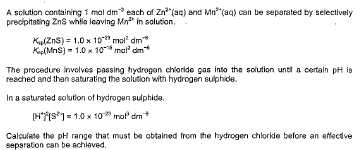Hi UltimaOnline,

For this question, I am more interested in the mechanics of the experiment rather than the answer.

If I had understood this procedure correctly, it is the part where “saturating the solution with hydrogen sulphide” that is providing the S2- ions which is used to precipitate ZnS, followed by MnS.

A: Now, what is the purpose of passing HCl into the solution prior to the saturation of H2S? My thinking is that it suppresses the dissociation of H2S (by LCP), thereby ensuring that only a very minute of S2- is present in the solution when it is added to prevent both precipitates from forming at the same time, given their very small Ksp values?

B: Next, how is the pH raised during the experiment to allow for the maximum effective precipitation (just right before MnS starts to precipitate)? My understanding for such reactions is that OH- ions will be supplied to decrease [H+] but this is not stated in the question. Is my understanding correct?

C: As I add more H2S into the solution, does the pH increase? I think it does because, the dissociation of H+ ions from H2S is too insignificant to counteract the decrease of [H+] from the dilution, hence resulting to a lower [H+] and thereby a higher pH.

• Originally posted by gohby:Hi UltimaOnline,

For this question, I am more interested in the mechanics of the experiment rather than the answer.

If I had understood this procedure correctly, it is the part where â€œsaturating the solution with hydrogen sulphideâ€� that is providing the S2- ions which is used to precipitate ZnS, followed by MnS.

A: Now, what is the purpose of passing HCl into the solution prior to the saturation of H2S? My thinking is that it suppresses the dissociation of H2S (by LCP), thereby ensuring that only a very minute of S2- is present in the solution when it is added to prevent both precipitates from forming at the same time, given their very small Ksp values?

B: Next, how is the pH raised during the experiment to allow for the maximum effective precipitation (just right before MnS starts to precipitate)? My understanding for such reactions is that OH- ions will be supplied to decrease [H+] but this is not stated in the question. Is my understanding correct?

C: As I add more H2S into the solution, does the pH increase? I think it does because, the dissociation of H+ ions from H2S is too insignificant to counteract the decrease of [H+] from the dilution, hence resulting to a lower [H+] and thereby a higher pH.

Yo Gohby!

A : Correct.

B : Correct.

C : Depends on the molarity of the H2S(aq) added. If H2S(g) is added, then the pH will still decrease (unless the solution is already saturated with H2S, then any additional H2S added to the solution will be forced to leave as H2S(g), so the pH won't change).
• Happy New Year, UltimaOnline! :)

Why does the pH decrease with the addition of H2S? Wouldn’t the mixing of a strong acid (HCl) with a weak acid (H2S) result to a lower [H+], thereby increasing the pH of the solution?

Now if the pH does decrease with the addition of H2S, and given that we need to increase the pH of the solution to point when MnS starts to precipitate by adding OH-, procedurally how does the experiment work – I assume that I add the H2S and OH- simultaneously? The question doesn’t offer a very clear picture tbh.

(P.S: I am deviating so much from the original question and I feel that I am being too scholastic… but I think a sound understanding of how this experiment works will aid one in arriving at the solution..)

• You too, Gohby! :)

For such experiments, you can saturate the soln first with H2S, then add OH- from eg. NaOH(aq) to control the pH to control precipitation. For this qn however, instead of adding OH-, the qn says you simply add H2S until sufficient S2- ions are present to precipitate out the less soluble metal sulfide. In other words, no OH- is added. You simply slowly add H2S until you get a ppt.

H2S is only acidic, thus even if it's a weak acidic, and a strong acid is already present, there's no way the pH can increase, unless the molarity is sufficiently low such that (as you previously mentioned) the dilution exceeds the proton dissociation.

Only when adding a basic (eg. S2-) or amphiprotic species (eg. HS-) to a strong acid, would the pH increase (as H+ is removed from soln by the amphiprotic species). But adding H2S (which is only acidic, not amphiprotic or basic) only serves to add more H+ into soln, thus decreasing pH further, but as stated previously, depending on (g) vs (aq), and depending on molarity of (aq) added.
• Hi UltimaOnline,

In a mixture of 0.1M of ethanoic acid (pKa 4.8 ) and 0.2M of bromic acid (pKa 8.7), the initial pH is 2.90. Why is it that the initial pH of the mixture is solely based on the [H+] from the ethanoic acid, and not from the bromic acid? Wouldn't the different [H+] from the bromic acid affect the overall [H+] in the mixture? (The question does not state the proportions of the acids in the mixture.)

Next, the 2 acids are titrated with NaOH. In drawing the titration curve, I understand that the base will always react with the stronger acid first, then the weaker acid after the neutralisation with the stronger acid. However, what is stopping the base from reacting with the weaker acid at the same time when it was reacting with the stronger acid?

Thank you! :)

• Originally posted by gohby:

Hi UltimaOnline,

In a mixture of 0.1M of ethanoic acid (pKa 4.8 ) and 0.2M of bromic acid (pKa 8.7), the initial pH is 2.90. Why is it that the initial pH of the mixture is solely based on the [H+] from the ethanoic acid, and not from the bromic acid? Wouldn't the different [H+] from the bromic acid affect the overall [H+] in the mixture? (The question does not state the proportions of the acids in the mixture.)

Next, the 2 acids are titrated with NaOH. In drawing the titration curve, I understand that the base will always react with the stronger acid first, then the weaker acid after the neutralisation with the stronger acid. However, what is stopping the base from reacting with the weaker acid at the same time when it was reacting with the stronger acid?

Thank you! :)

Yo Gohby,

First of all, the qn is erroneous. Bromic acid (Latin name) aka bromic(V) acid (Stock name) is a much stronger acid than ethanoic acid (Cambridge can ask H2 students to explain why). The qn was referring to hypobromous acid (Latin name) aka bromic(I) acid (Stock name), an acid weaker than ethanoic acid.

In which case, yes the pH of the soln is mostly controlled by the significantly stronger ethanoic acid. This is because as predicted by Le Chatelier's principle, the proton dissociation of the weaker acid is suppressed by the shifting of position of equilibrium by larger Ka of the stronger acid.

In other words, in excess H+ (from the stronger acid), the position of equilibrium shifts back to the undissociated HOBr. In this shifting of equilibrium position, Qa > Ka, (note that Ka value doesn't change because Ka is an equilibrium constant at constant temperature), hence position of equilibrium shifts from RHS, ie. BrO- + H+, to the LHS, ie. HOBr, until equilibrium is re-estabilished, ie. Qa = Ka.

But you're right that the weaker acid does still affect pH, and at Uni level Chemistry, may (depending on the magnitude of the difference in Ka values) still have to be taken into account for a more accurate calculation. For A level purposes, Cambridge will always choose acids that allow the A level approximation to be chemically and mathematically valid.

Nothing is stopping the base from reacting with the weaker acid at the same time when it was reacting with the stronger acid, but because this is an equilibrium, hence if the base deprotonates the weaker acid, equilibrium will take over to transfer another proton from the stronger acid to the weaker acid, concordantly and consequently in effect, it's as if the base deprotonated the stronger acid in the first place. Hence mathematically, we simply calculate based on deprotonating the stronger acid directly.

U're welcome ;)
• Q1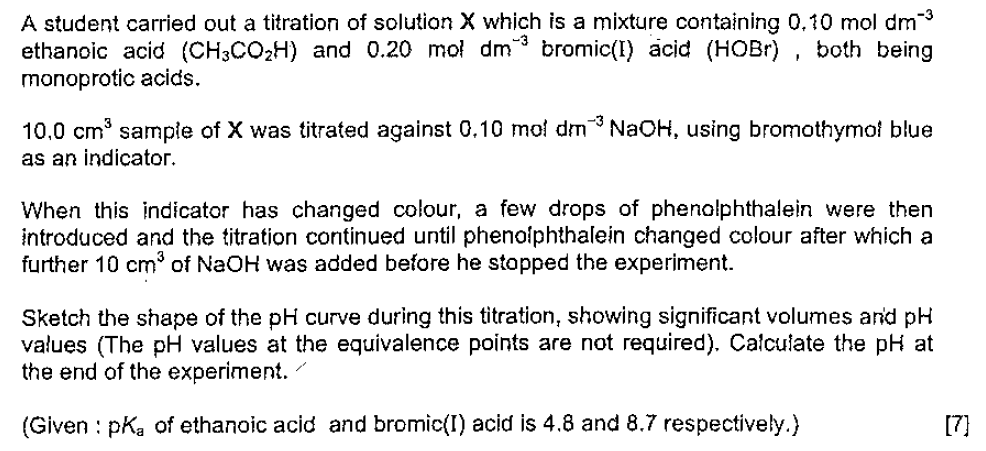As a follow up to the previous question, the question was correct as I omitted the (I) in the bromic (I) acid.

The answer indicates the precise volumes of sodium hydroxide required for during the 2 equivalence points as 10cm³ and 30cm³. However, wouldn’t that mean we have to assume that the mixture contains an equal volume of ethanoic acid and bromic (I) acid? Is this a reasonable assumption to make in light of the information provided?

Q2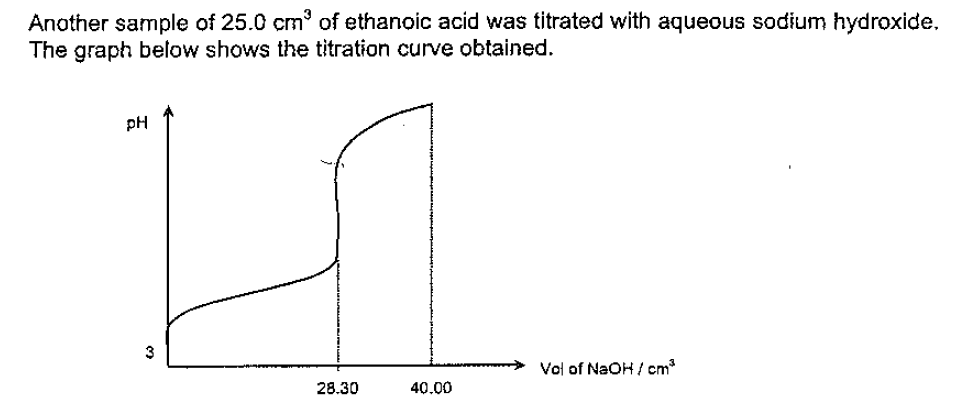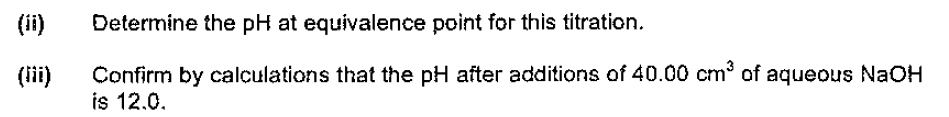For part (ii) my pH at equivalence point is 8.61 but the answer suggests 8.58. Did I overlook something? As for (iii), I am only able to get a pH of 11.946 (5sf).

I carried out my calculations using the exact values from the calculator. Alternatively, I would write 5sf for my intermediate workings and round it up to 3sf for the final answer. Both of them do not yield a pH of 12.0. If my calculations are correct, should I reduce the number of significant figures just to “confirm” the value of 12.0?

Q3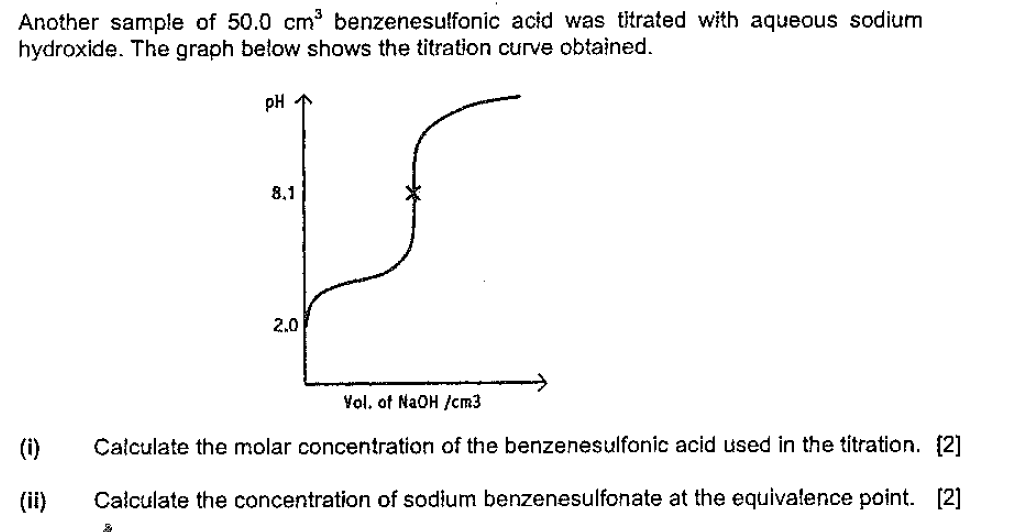(Ka of acid = 5.9x10^-4M)

I reckon this is a blind spot on my part, but using the formula Ka ≈ [H+]²/[acid] yields an answer of 0.169M instead of 0.179M as suggested by the answer. As for (ii) the answer suggests 0.0937M - I drew the ICE table but was unable to obtain 0.0937M exact - similar to the problem above. Is there something wrong with my calculation method and my degree of accuracy?

Thank you once again, UltimaOnline :)

• Originally posted by gohby:

Q1As a follow up to the previous question, the question was correct as I omitted the (I) in the bromic (I) acid.

The answer indicates the precise volumes of sodium hydroxide required for during the 2 equivalence points as 10cmÂ³ and 30cmÂ³. However, wouldnâ€™t that mean we have to assume that the mixture contains an equal volume of ethanoic acid and bromic (I) acid? Is this a reasonable assumption to make in light of the information provided?

Q2For part (ii) my pH at equivalence point is 8.61 but the answer suggests 8.58. Did I overlook something? As for (iii), I am only able to get a pH of 11.946 (5sf).

I carried out my calculations using the exact values from the calculator. Alternatively, I would write 5sf for my intermediate workings and round it up to 3sf for the final answer. Both of them do not yield a pH of 12.0. If my calculations are correct, should I reduce the number of significant figures just to â€œconfirmâ€� the value of 12.0?

Q3(Ka of acid = 5.9x10^-4M)

I reckon this is a blind spot on my part, but using the formula Ka â‰ˆ [H+]Â²/[acid] yields an answer of 0.169M instead of 0.179M as suggested by the answer. As for (ii) the answer suggests 0.0937M - I drew the ICE table but was unable to obtain 0.0937M exact - similar to the problem above. Is there something wrong with my calculation method and my degree of accuracy?

Thank you once again, UltimaOnline :)

Q1. The question has to be interpreted to mean the stated molarities of 0.1M and 0.2M are not before mixing, but after mixing.

Q2. Question is erroneous (if taken as a new, unrelated qn to Q1) because the molarity of *either* NaOH or CH3COOH acid has to be given, but isn't.

(ii) If either molarity is given, then you can work out the moles, hence new molarity of CH3COO- ions, then applying Kb, you can work out [OH-] hence pOH hence pH.

(iii) An ICF (not ICE) table should be done to obtain the Final (the F in ICF) moles of OH- in excess. As predicted by Le Chatelier's principle, you can ignore the negligible hydrolysis of the much weaker base CH3COO-, and calculate pH based on the new moles, hence molarity, of excess OH- in solution.

Your values are close enough to the given answer. Cambridge (depending on question and required working) may accept a reasonable accuracy of 2 sig fig. The inaccuracy may lie with whoever set this qn, no worries. Just ensure your students have the correct underlying concepts.

Q3. Question is erroneous. Benzenesulfonic acid is considered a strong acid with a much larger Ka value than the question provides. To put that aside, let's assume we change the qn to a weak acid with the Ka you provided. Then both the answers you gave (ie. both your own and the stated answers) appears to be wrong. If you want to discuss this further, can you show detailed mathematical working on how you arrived at your answer? And what is the source of this qn? Which JC, which year?

A further problem arises : the assumption that has to be made at A levels (which Cambridge allows so as to not require a graphing calculator for H2 Chem) that equilibrium molarity can be approximated to initial molarity because change in molarity is much smaller than initial molarity for weak acid, no longer holds true for this question. Because the initial [H+] is too large as the initial pH is too acidic. As such, graphing calculator to solve quadratic equation is required, and hence this question will not be asked at A levels.

For (ii), from Ka of HA, calculate Kb of A-. Hence from Kb formula, plugging in the [OH-] (calculated from pH read from graph), you can work out the [A-], which solves the question. If you did this, ie. your approach is correct, then no worries about the mathematical discrepancy. It may likely be the question setter's fault, not yours.
• Hi UltimaOnline,

Q2 (YJC Prelims 04 P3): Urgh my bad. The Ka of ethanoic acid is given as 1.8x10^-5M. It was embedded in part (a) of the same question which was unrelated to part (b), save for this Ka value, so I extracted part (b) in order not to clutter my question, but I forgot to include the Ka value from embedded in part (a).

I think these are standard questions; normally I am not very concerned about slight deviations with the answers. However this question specifically required the confirmation that the pH is 12.0, and I could only get 11.946 (and 8.61 for part (ii)).

For Q3, this is a HCJC Prelims 02/P3 question. My workings are as follows:

Given that the initial pH of the acid is 2.0, so [H+] = 10^-2. I used the formula Ka ≈ [H+]²/[initial acid], i.e. 5.9x10^-4 = [10^-2]²/ [initial acid]. So I found [initial acid] to be 0.16949M (5sf).

My detailed workings for part (b) are as follows:

Kb = 10^-14/5.9x10^-4 ≈ [OH-]²/[sodium benzenesulfonate]

Since pH at equivalence point is 8.1, [OH-]² = 1.5849x10^-12. Hence [sodium benzenesulfonate] = 0.093509M. However the answer provided was 0.0937M.

• Originally posted by gohby:

Hi UltimaOnline,

Q2 (YJC Prelims 04 P3): Urgh my bad. The Ka of ethanoic acid is given as 1.8x10^-5M. It was embedded in part (a) of the same question which was unrelated to part (b), save for this Ka value, so I extracted part (b) in order not to clutter my question, but I forgot to include the Ka value from embedded in part (a).

I think these are standard questions; normally I am not very concerned about slight deviations with the answers. However this question specifically required the confirmation that the pH is 12.0, and I could only get 11.946 (and 8.61 for part (ii)).

For Q3, this is a HCJC Prelims 02/P3 question. My workings are as follows:

Given that the initial pH of the acid is 2.0, so [H+] = 10^-2. I used the formula Ka â‰ˆ [H+]Â²/[initial acid], i.e. 5.9x10^-4 = [10^-2]Â²/ [initial acid]. So I found [initial acid] to be 0.16949M (5sf).

My detailed workings for part (b) are as follows:

Kb = 10^-14/5.9x10^-4 â‰ˆ [OH-]Â²/[sodium benzenesulfonate]

Since pH at equivalence point is 8.1, [OH-]Â² = 1.5849x10^-12. Hence [sodium benzenesulfonate] = 0.093509M. However the answer provided was 0.0937M.

Ah ok Gohby, no worries then, your working is fine. It's likely that the question setter used a slightly different (eg. 3sf instead of the given 2sf) value for Ka, in his/her own calculations. Another possibility could be related to my next point.

What I've pointed out earlier, still holds. Benzenesulfonic acid should have a much larger Ka (and Cambridge can give a few Ka values of other acids and ask the student to deduce, with reasons, which is the correct Ka of benzenesulfonic acid). And if the initial [H+] is so high, the approximation is no longer valid. In other words, you must use the formula Ka = ([H+] x [A-]) / (initial [HA] - [H+]).

Perhaps this is what the question setter did. You can try it out to see if the answer is closer to the question setter's answer (and explain to your students why this is a more correct working ; ie. because the proton dissociation is no longer negligible compared to the initial molarity of the acid, hence the approximation is no longer mathematically valid to 3 sf).

No prob! ;)
• Originally posted by UltimaOnline:

Ah ok Gohby, no worries then, your working is fine. It's likely that the question setter used a slightly different (eg. 3sf instead of the given 2sf) value for Ka, in his/her own calculations. Another possibility could be related to my next point.

What I've pointed out earlier, still holds. Benzenesulfonic acid should have a much larger Ka (and Cambridge can give a few Ka values of other acids and ask the student to deduce, with reasons, which is the correct Ka of benzenesulfonic acid). And if the initial [H+] is so high, the approximation is no longer valid. In other words, you must use the formula Ka = ([H+] x [A-]) / (initial [HA] - [H+]).

Perhaps this is what the question setter did. You can try it out to see if the answer is closer to the question setter's answer (and explain to your students why this is a more correct working ; ie. because the proton dissociation is no longer negligible compared to the initial molarity of the acid, hence the approximation is no longer mathematically valid to 3 sf).

No prob! ;)

Hi UltimaOnline,

Your explanation was spot on! For 3(i) when I calculate the pH without the approximation I got the answer to be 0.179M. :)

However, how do we ascertain if the [H+] is sufficiently high for which the approximation would not be valid? Separately, when we calculate the pH at the equivalence point are there circumstances where we cannot approximate the [salt] as the intial concentration of the salt as the dissociation is deemed to be significant?

• Originally posted by gohby:

Hi UltimaOnline,

Your explanation was spot on! For 3(i) when I calculate the pH without the approximation I got the answer to be 0.179M. :)

However, how do we ascertain if the [H+] is sufficiently high for which the approximation would not be valid? Separately, when we calculate the pH at the equivalence point are there circumstances where we cannot approximate the [salt] as the intial concentration of the salt as the dissociation is deemed to be significant?

Nice :)

Actually there are 2 reasons why approximation should not be used for this question (as well as a similar Cambridge question asked over 10 years ago).

Firstly, as I said, [H+] in Change is not << initial [HA], hence equilibrium [HA] can't be approximated to initial [HA]. That pH is 2.0 (furthermore by the structure benzenesulfonic acid, you can expect it to be a strong acid) should sound a warning bell that [H+] won't be small enough for the approximation to be valid. Mathematically, initial [HA] should be at least 100x larger than [H+] dissociated for the approximation to be valid to 3 sig fig.

Secondly, the practical reason why Cambridge allows the approximation is because H2 Chem students are not expected to have graphing calculators with them. And nobody is expected to solve quadratic equations without a graphing calculator or app these days. And notice that for this question (as well as the Cambridge question over 10 years ago), there was no need to solve a quadratic equation (since you only get x, not x^2). Hence, there is no excuse (eg. "I don't have a graphing calculator!") to use an approximation in the first place, so full credit would only be given if the correct, non-approximation working was used.

Regarding salt hydrolysis, same thing. Treat it as any weak base. If the [A-] used up by hydrolysis (indicated by Kb value) is too large to approximate equilibrium molarity back to initial molarity, then graphing calculator has to be used to solve for quadratic. In this case, Kb value is so small, the [A-] used up hydrolysis will be much smaller than initial, hence approximation is valid, graphing calculator is not required.
• Originally posted by gohby:

Q3(Ka of acid = 5.9x10^-4M)

I reckon this is a blind spot on my part, but using the formula Ka ≈ [H+]²/[acid] yields an answer of 0.169M instead of 0.179M as suggested by the answer. As for (ii) the answer suggests 0.0937M - I drew the ICE table but was unable to obtain 0.0937M exact - similar to the problem above. Is there something wrong with my calculation method and my degree of accuracy?

Thank you once again, UltimaOnline :)

Hi UltimaOnline,

Apologies for digging out this post but I realised some of the discrepancies with sf arose because I did the approximation method to negate the dissociation of the acid when I shouldn't have done so. Hence I am relooking at other questions where there are discrepancies wrt the accuracy of the answers.

For 3(ii) (Ka of acid = 5.9x10^-4M), here are my workings but I think I have missed out on an aspect which would explain the disparity between my answer and the suggested answer of 0.0937M. Could you see if my workings/assumptions are in order?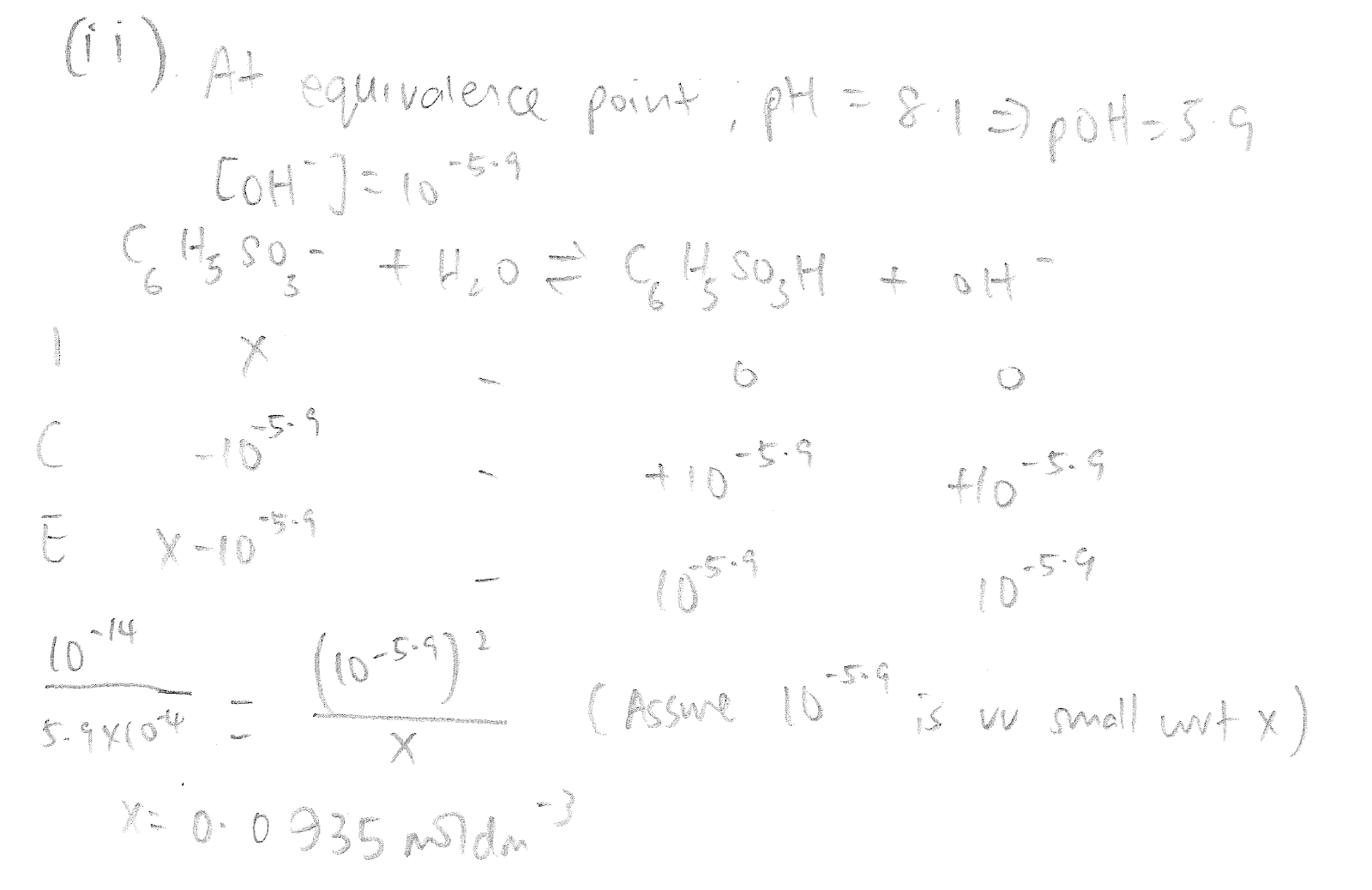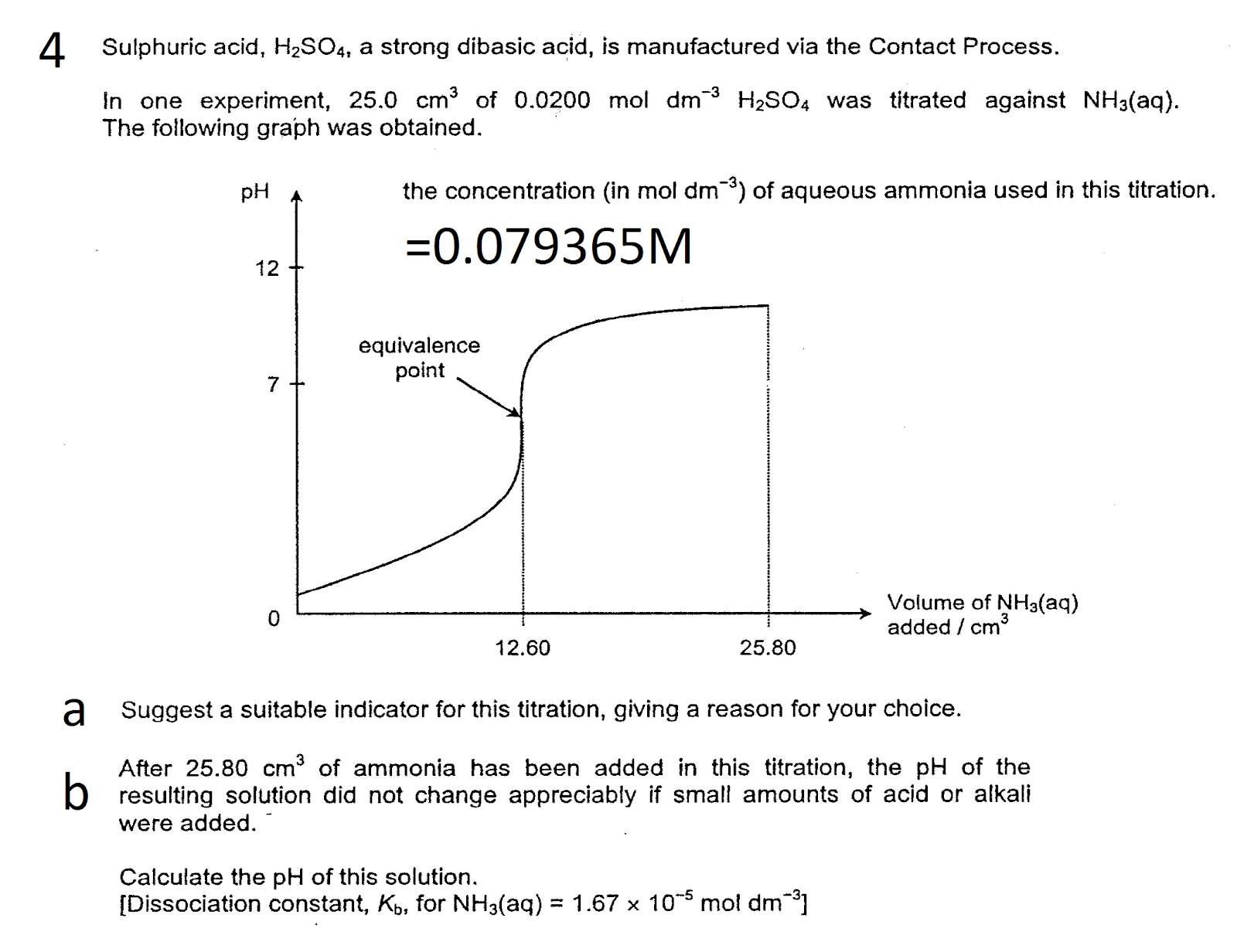For 4a would it be wrong if I suggest litmus as a suitable indicator? The answer suggested methyl orange but I doubt the working range of methyl orange coincides with the rapid pH change over the equivalence point.

As for 4b, my workings are as follows: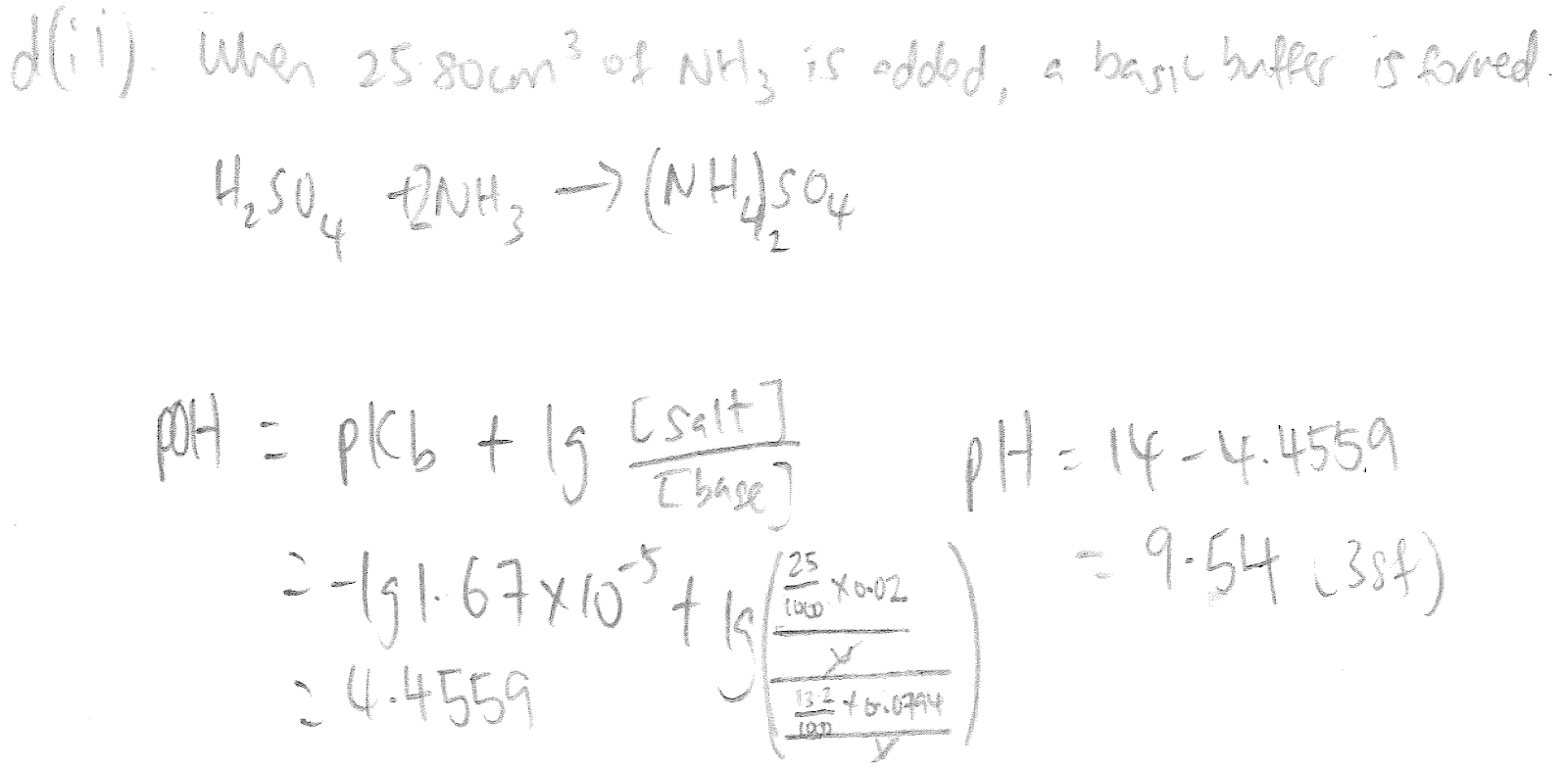The answer suggested that the pH is 9.24 - is there something wrong with my workings?

Thank you :)

• Originally posted by gohby:

Hi UltimaOnline,

Apologies for digging out this post but I realised some of the discrepancies with sf arose because I did the approximation method to negate the dissociation of the acid when I shouldn't have done so. Hence I am relooking at other questions where there are discrepancies wrt the accuracy of the answers.

For 3(ii) (Ka of acid = 5.9x10^-4M), here are my workings but I think I have missed out on an aspect which would explain the disparity between my answer and the suggested answer of 0.0937M. Could you see if my workings/assumptions are in order?For 4a would it be wrong if I suggest litmus as a suitable indicator? The answer suggested methyl orange but I doubt the working range of methyl orange coincides with the rapid pH change over the equivalence point.

As for 4b, my workings are as follows:The answer suggested that the pH is 9.24 - is there something wrong with my workings?

Thank you :)

Hi Gohby,

For Q3 your working is fine. You can check your assumption of mathematical validity by plugging in the values after you get your final answer. Is initial molarity >> change, such that equilibrium molarity can be approximated back to initial molarity to 3 sf? Since 0.0935 - (10^-5.9) = 9.3499 x 10^-2 = 0.0935 (to 3 sf), hence your approximation is mathematically valid.

For Q4a, litmus changes colour at pH 7, since pH at equivalence point for this titration is approx 5 (based on graph), hence litmus won't be acceptable. But methyl orange (which changes color at pH 3.8) won't be acceptable either. A better indicator would be methyl red (which changes color at pH 5).

For Q4b, first of all the question is wrong to say H2SO4 is a strong diprotic acid, the 1st proton dissociation is certainly strong, but the 2nd proton dissociation is slightly weak. But in this case, because NH3 is a significantly stronger Bronsted-Lowry base than SO4 2-, hence the final answer for this question remains the same (admittedly, correctly considering the strengths of the 1st vs 2nd proticities of H2SO4 is somewhat beyond the H2 syllabus, and Cambridge will give additional guidance in the question if it expects students to take this into consideration).

I can't say for sure what's wrong with your working, but this is how I'd recommend students to approach this question. Since the approximation for H2SO4 as a strong diprotic acid is acceptable for this qn, the equation should be written as H+ + NH3 --> NH4+. Doing the ICF (Initial Change Final) table for the Bronsted-Lowry proton transfer reaction, the final moles are 0 (for H+, not H+ in soln, but H+ from H2SO4), 0.0010476 (for NH3) and 0.001 (for NH4+). Plugging these moles (no need to convert moles into molarities, since molarities/molarities in the same solution = moles/moles) into the Henderson-Hasselbalch equation, ie. pH = pKa + log ( [base] / [acid] ), the pH will be calculated to be 9.2429, ie. 9.24 (to so-called 3 sf, for to be precise 9.24 is to 2 sf, as due to the log function, only the digits behind the decimal point are counted as significant figures, but apparently this isn't taught in Singapore JCs, and Cambridge will accept the 9.24 (2 sig fig) answer, unless otherwise specified by the qn).